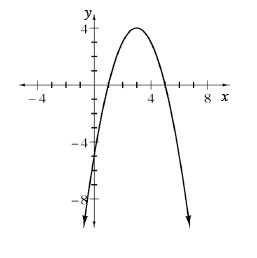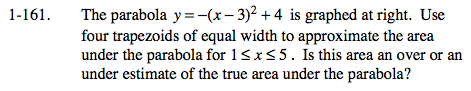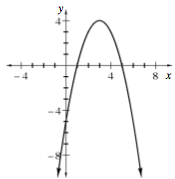### Home > CALC > Chapter 1 > Lesson 1.4.2 > Problem1-161

1-161.

The parabola y = (x − 3)2 + 4 is graphed below. Use four trapezoids of equal width to approximate the area under the parabola for 1 ≤ x ≤ 5. Is this area an over or an under estimate of the true area under the parabola? Explore this using the Estimating Area Under a Curve eTool (Desmos). Homework Help ✎Use the eTool below to visualize the flag.
Click the link at right for the full version of the eTool: Estimating Area Under a Curve eTool

$\text{Area}=\frac{1}{2}(b_1+b_2)h$

The height of each trapezoid is 1. The bases are determined by the function.